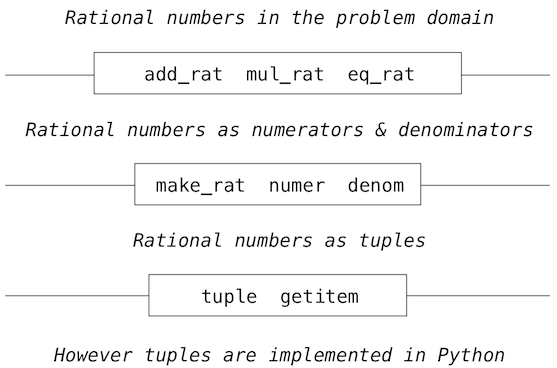# 2.2 数据抽象

## 2.2.1 示例：有理数的算术

``````<numerator>/<denominator>
``````

``````>>> 1/3
0.3333333333333333
``````

``````>>> 1/3 == 0.333333333333333300000  # Beware of approximations
True
``````

• `make_rat(n, d)`返回分子为`n`和分母为`d`的有理数。
• `numer(x)`返回有理数`x`的分子。
• `denom(x)`返回有理数`x`的分母。

``````>>> def add_rat(x, y):
nx, dx = numer(x), denom(x)
ny, dy = numer(y), denom(y)
return make_rat(nx * dy + ny * dx, dx * dy)
>>> def mul_rat(x, y):
return make_rat(numer(x) * numer(y), denom(x) * denom(y))
>>> def eq_rat(x, y):
return numer(x) * denom(y) == numer(y) * denom(x)
``````

## 2.2.2 元组

``````>>> (1, 2)
(1, 2)
``````

``````>>> pair = (1, 2)
>>> pair
(1, 2)
>>> x, y = pair
>>> x
1
>>> y
2
``````

``````>>> pair
1
>>> pair
2
``````

Python 中的元组（以及多数其它编程语言中的序列）下标都以 0 开始，也就是说，下标 0 表示第一个元素，下标 1 表示第二个元素，以此类推。我们对这个下标惯例的直觉是，下标表示一个元素距离元组开头有多远。

``````>>> from operator import getitem
>>> getitem(pair, 0)
1
``````

``````>>> def make_rat(n, d):
return (n, d)
>>> def numer(x):
return getitem(x, 0)
>>> def denom(x):
return getitem(x, 1)
``````

``````>>> def str_rat(x):
"""Return a string 'n/d' for numerator n and denominator d."""
return '{0}/{1}'.format(numer(x), denom(x))
``````

``````>>> half = make_rat(1, 2)
>>> str_rat(half)
'1/2'
>>> third = make_rat(1, 3)
>>> str_rat(mul_rat(half, third))
'1/6'
'6/9'
``````

``````>>> from fractions import gcd
>>> def make_rat(n, d):
g = gcd(n, d)
return (n//g, d//g)
``````

``````>>> str_rat(add_rat(third, third))
'2/3'
``````

## 2.2.3 抽象界限## 2.2.4 数据属性

• 如果一个偶对`p``x``y`构造，那么`getitem_pair(p, 0)`返回`x``getitem_pair(p, 1)`返回`y`

``````>>> def make_pair(x, y):
"""Return a function that behaves like a pair."""
def dispatch(m):
if m == 0:
return x
elif m == 1:
return y
return dispatch
>>> def getitem_pair(p, i):
"""Return the element at index i of pair p."""
return p(i)
``````

``````>>> p = make_pair(1, 2)
>>> getitem_pair(p, 0)
1
>>> getitem_pair(p, 1)
2
``````